## FRUIT PICKING

### SUBTRACTING WITHIN 20

Emerge subtraction strategies and models to efficiently subtract numbers within 20.

## Intentionality & Unit Overview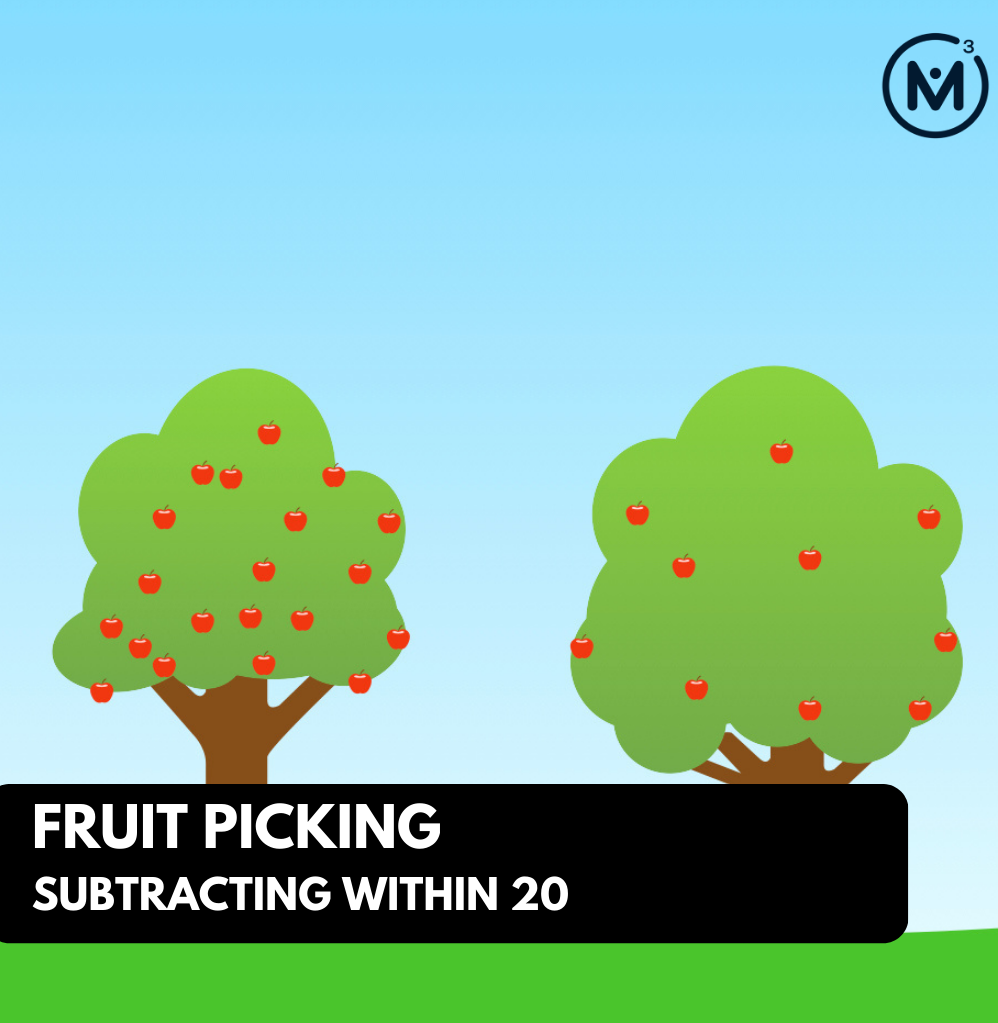### Access each lesson from this unit using the navigation links below

Students will explore subtraction in a context by taking numbers away from ten. A connection can be made to the combinations that make ten.

## Intentionality…

In this task, students will explore the combinations of ten through a subtraction focus. The relationship between addition and subtraction can be highlighted as students recognize that knowing the addition fact will assist with the subtraction fact.

Big ideas of the unit:

• Understanding hierarchical inclusion allows for flexible composing and decomposing of numbers
• Numbers can be decomposed by separating a whole into two or more parts
• Subtraction names the missing part in terms of the whole
• Different subtraction situations will elicit different strategies
• Number relationships provide the foundation for strategies to help students remember basic facts
• Addition and subtraction are related in that addition names the parts in the whole and subtraction uses the whole to find a missing part.
• Subtraction can be used in either take away, comparison, or missing addend situations.

## What Do You Notice? What Do You Wonder?

Show students the following video:

What do you notice?
What do you wonder?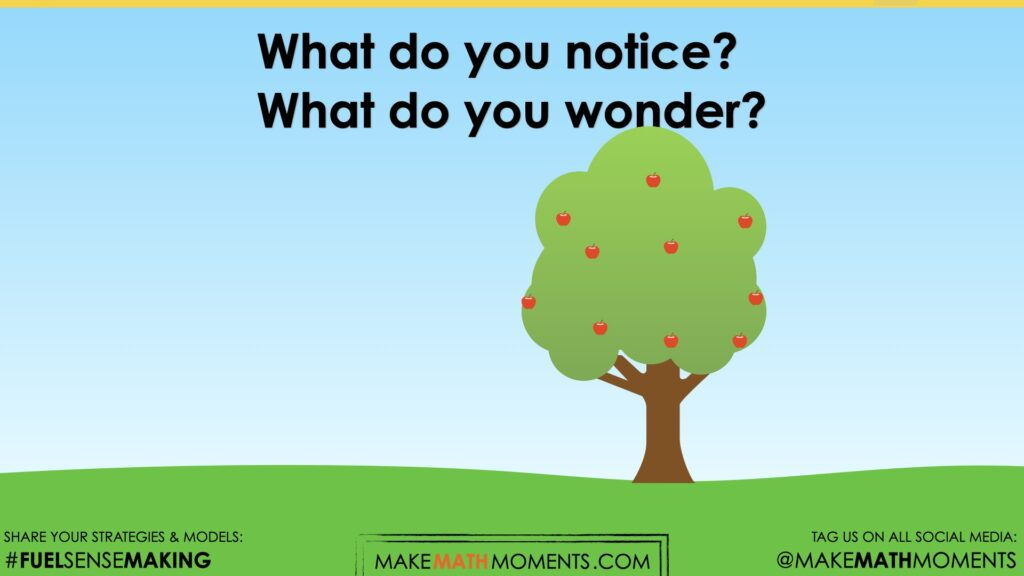Give students 60 seconds (or more) to do a rapid write on a piece of paper.

Then, ask students to share with their neighbours for another 60 seconds.

Finally, allow students to share with the entire group.

Some of the noticing and wondering that came up in a class recently included:

• I notice there are trees
• I notice there are apples
• I notice there are 10 apples on the tree
• I wonder this is an apple orchard
• I wonder if we can eat the apples

## Estimation: Prompt

Next, share the following visual prompt briefly and ask the following question:

Each tree in the orchard has a different amount of apples on it. Every tree has 10 or more apples. None of the trees have more than 20 apples. How many apples could be on the trees?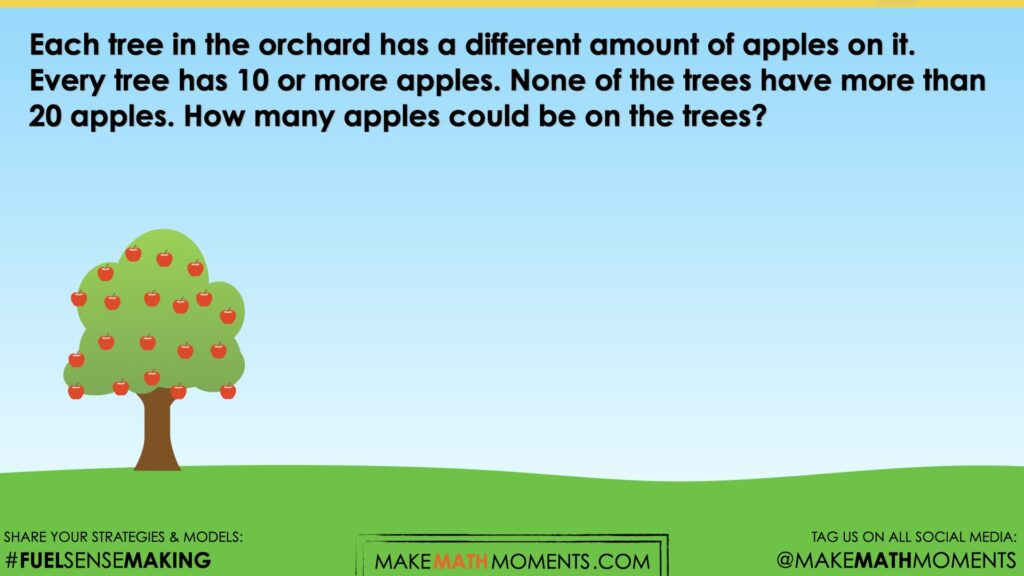## Estimation: Reveal

Share the following video and or image revealing how the floors in the parking garage have been labelled.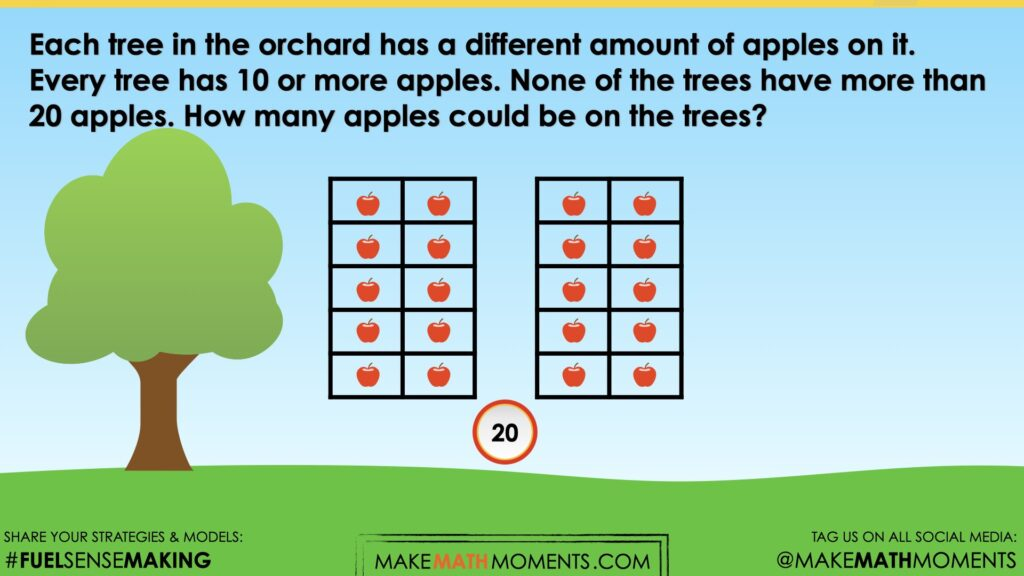## Crafting A Productive Struggle: Prompt

Since you have already taken some time to set the context for this problem and student curiosity is already sparked, we have them in a perfect spot to help push their thinking further and fuel sense making.

Share the following prompt.

One of the trees has 10 apples on it. The apple picker came and picked 6 apples.

How many apples are still on the tree?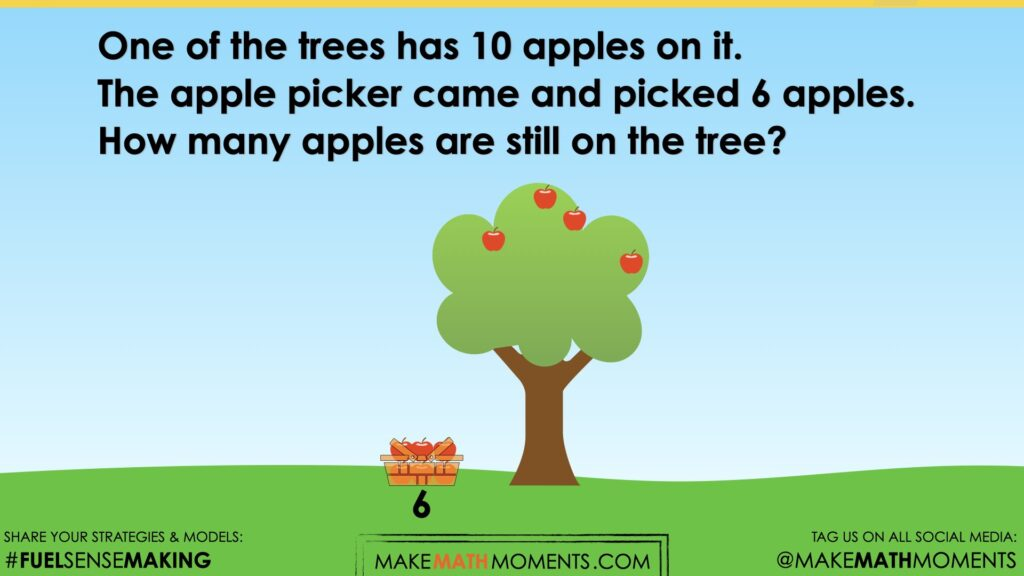## While Students Are Productively Struggling…

Monitor student thinking by circulating around the room and listening to the mathematical discourse. Select and sequence some of the student solution strategies and ask a student from the selected groups to share with the class from:

• most accessible to least accessible solution strategies and representations;
• most common misconceptions;
• most common/frequent to least common/frequent representations; or,
• choose another approach to selecting and sequencing student work.

The strategies you might see students use include:

• Direct Modeling and counting all
• Counting back

Have students share their reasoning for determining the number of apples still on the tree. Discuss their thinking during your consolidation to build off of their current prior knowledge and understanding rather than “fixing” or “funneling” student thinking to a representation and justification that does not connect to their understanding.

Assessment:

This checklist can be used for tracking formative assessment as students are working. The information collected can be used to form whole group, small group or one-to-one support models.

 Early strategy Direct modeling and counting all Students will count the initial value, count the amount added then count all of the amounts. Example: Count 1: 8 blocksCount 2: 5 blocksCount 3: 8 blocks and the 5 blocks to know 13 blocks in all Pre-cursor skills Count backwards from various points Watch for students that need to count forwards in order to start counting backwards.  You may hear a student that is counting back from 8, begin their count “1, 2, 3, 4, 5, 6, 7, 8” then start counting back. Near Numbers Student understanding of the numbers one or two more/less is important in the count sequence Count Back strategy Students are holding one number in their head and continuing to count backwards and track their count Count Up Students are finding the difference/space between in the numbers by adding up from the lower number to the higher number Strategic and efficient strategy (e.g. Known facts, Down and Under Ten, etc) Students may start to use known addition facts to support their understanding of subtraction. Students may also use benchmark numbers like 10 when subtracting. The Down and Under Ten strategy is effective when subtracting from a teen number.

## Student Approach #1: Direct Modeling and Counting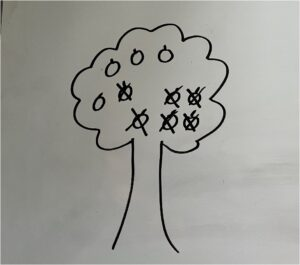I drew the tree with 10 apples on it. I crossed off 6 of the apples and counted the ones that were left. There was 4 of them. So there are 4 left on the tree.

## Student Approach #2: Using fingers to Count Back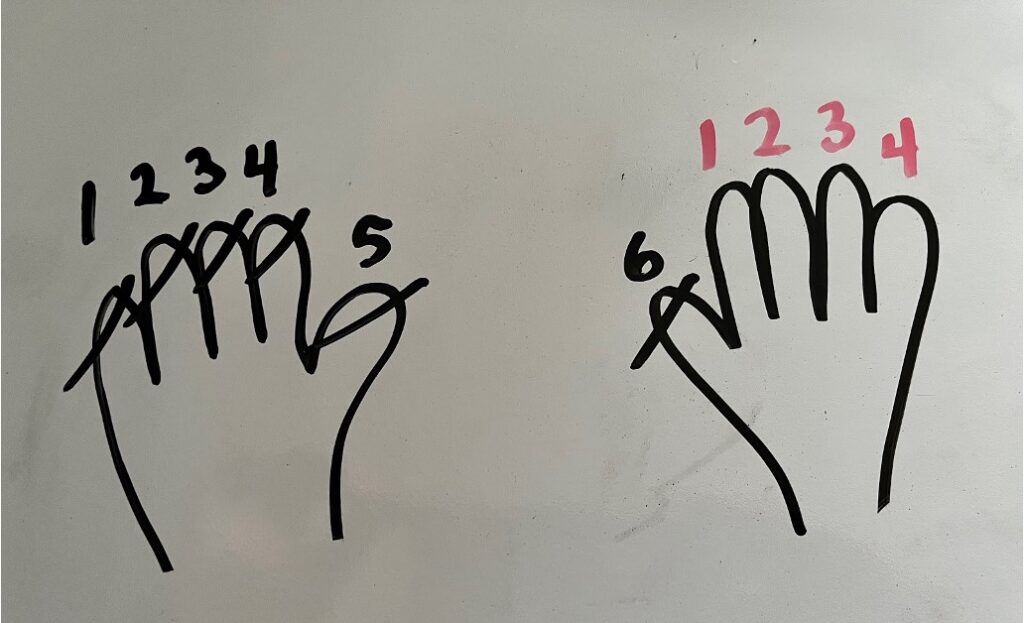I put up 10 fingers to pretend that they were apples. I put down 6 of the fingers. There were still 4 fingers up. So there are 4 apples left on the tree.

## Student Approach #3: Known Addition Fact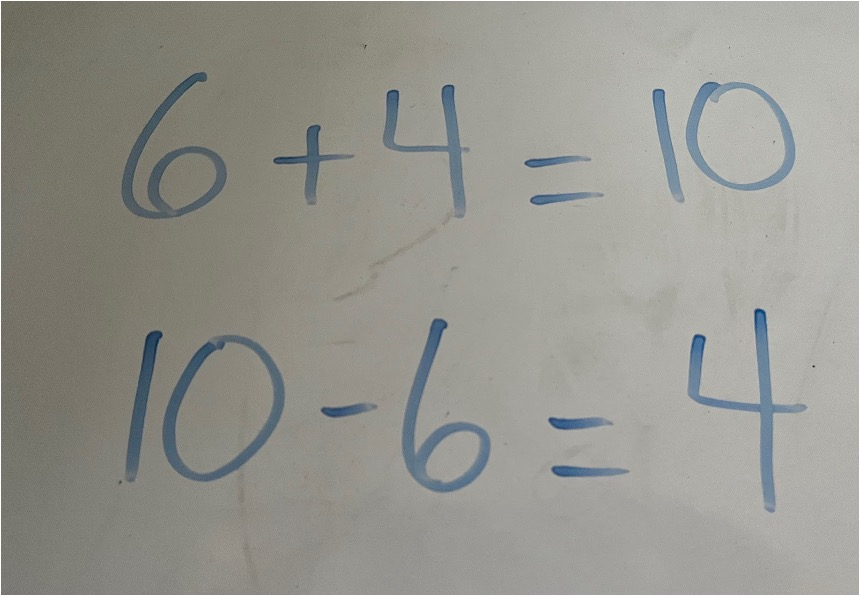I know that 6 + 4 is 10, so if there were 10 apples on the tree, and 6 were picked, then there must be 4 left because 10 – 6 is 4.

## Consolidation

The big idea in this introductory lesson is to explore the combinations of ten from the subtraction lense. Students should start to make the connections between the known addition facts to assist with the opposite subtraction fact.

During consolidation, consider using a part whole mat to display the thinking:

 10 6 ?

Throughout this unit, we will be using the combinations of ten when subtracting from a teen number. Students will subtract to get to a 10, then they will need to use their combinations of ten to help them subtract the remaining amount. This strategy is called “Down Under Ten”, it will be explored more in Day 5.

Subtraction within 10 is a foundational skill for student understanding. Students need exposure to subtraction to develop the relationship between numbers. It is possible that students have spent a lot more time working with addition strategies but the consolidation is a great time to foster the connection between the addition facts and their subtraction opposite.  Student practice of decomposing and composing numbers (possibly using a part whole mat as a visual) will increase flexibility of the subtraction strategies.

At this stage, it is possible that students are using manipulatives or their fingers to completely model the concept. Since the numbers are within 10, the fingers become a very obvious tool to use.

It is also noteworthy to discuss that counting up is often a much more efficient strategy than counting back. The counting back strategy is a crucial first step in the subtraction continuum. As students are exposed to bigger numbers, more efficient strategies will be important to explore. It is important that students understand that counting up and taking away are two different subtraction strategies.

Symbolism:

To introduce symbolism, it can be stated that it is a way to record thinking to a contextual problem. There will be varying exposure to the subtraction symbol in a classroom but students can have an understanding of a subtraction context at a very early age. As early a grade 1, students should start to engage with the subtraction symbol.

For example, “We knew that were 10 apples were on the tree, 6 apples were picked. We figured out that there were 4 apples left. We can write that using special symbols:

10 – 6 = 4  The subtraction symbol should be read as “minus” or “subtract” rather than “take away” to eliminate confusion of the different meanings of subtraction.

Facilitator Notes

To continue supporting students:

• Change the amount of apples that are picked from the tree from 6 to another number within 10
• Change the number of apples that are starting on the tree
• Act out the problem by changing the context to students in the classroom. E.g.There are 7 students on the carpet, 5 of them went back to their seats. How many students are still on the carpet?

## Reflect and Consolidation Prompts

Provide students an opportunity to reflect on their learning by offering these consolidation prompts to be completed independently.

### Consolidation Prompt #1:

How are 10 – 6 = 4 and 6 + 4 =10 the same? How are they different?

### Consolidation Prompt #2:

10 – 3 = ?

Write a math problem that would match this question.

We suggest collecting this reflection as an additional opportunity to engage in the formative assessment process to inform next steps for individual students as well as how the whole class will proceed.

## Educator Discussion Area

Login/Join to access the entire Teacher Guide, downloadable slide decks and printable handouts for this lesson and all problem based units.

## Explore Our 60+ Problem Based Units

This Make Math Moments Lesson was designed to spark curiosity for a multi-day unit of study with built in purposeful practice, number talks and extensions to elicit and emerge strategies and mathematical models.

Dig into our other units of study and view by concept continuum, grade or topic!